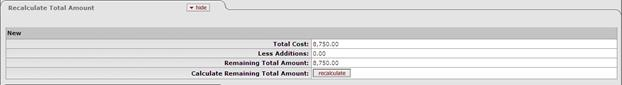﻿ Recalculate Total Amount Tab
###### Recalculate Total Amount Tab

This tab displays the current cost of the asset, any deductions, and the remaining total amount. It also allows the user to recalculate the total amount after making changes to the document.Recalculate Total Amount tab definition

 Title Description Total Cost Total cost of the asset from all payments posted to the asset. Less Additions Total cost of all new asset that will be reduce the cost of the original asset. Remaining Total Amount Amount left as the final cost of the original asset. Calculate Remaining Total Amount This button allows the user to recalculate the cost of the asset and display the amount remaining for the asset.

More: